2. Fields of Rest-Motion (A Case of Harmonic Oscillations of a Material Point)

L. G. Kreidik (translation from Russian T. S. Kortneva and G. P. Shpenkov)

2.1. A measure of non-occurrence of a moving object

We will consider the problem of description of motion from the point of view of dialectics for harmonic oscillation of a material point as an example: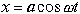. (2.9)

The displacement x is a measure of location of an object at a certain point of space. Two pairs of opposite points are distinguished in the harmonic oscillation. They are two points of unstable equilibrium and two points of stable equilibrium, located at one point of space. At certain moments, the object stops at the points of unstable equilibrium and it is therefore possible to say that it only occurs at these points. The object passes the points of stable equilibrium with a maximum velocity, therefore we can say that it does not occur at the equilibrium points.

With this description, one can state that at the other points the object occurs and does not occur simultaneously. A measure of non-occurrence can be negation of the displacement X, both quantitatively and qualitatively. A cosine displacement is negated by a sine displacement, therefore the quantity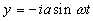, (2.10)

which negates X at least at the points of stable and unstable equilibrium, will be taken as the simplest measure of non-occurrence. It becomes zero, whenever x has extreme values and is an extremum, whenever x is zero.

This choice of the non-occurrence measure y will be revealed as introducing new relevant concepts, appropriate calculations and consequences from them.

2.2. Harmonic displacement from the viewpoint of dialectics

Combining both occurrence X and non-occurrence y measures into one binary harmonic displacement, we have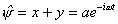. (2.11)

The harmonic displacement is an oppositus-measure which express simultaneous occurrence and non-occurrence of the object at any point of the trajectory. The first component of the displacement, i.e. a displacement of stay, expresses the potential aspect of the process, its rest. The second component, a displacement of non-stay, describes the kinetic aspect of the process, its motion.

Thus, the harmonic displacement is a potentially kinetic or kinematic displacement. Further, the objective contradiction "potentially kinetic" we will call very often by one name "kinematic".

For comparison of harmonic oscillation with other kinds of motion, the notion of specific displacement is very convenient: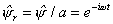, (2.12)

It is related to the displacement by the simple relation: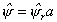. (2.13)

This (2.13) relation between the linear and specific displacements will be extended to any parameters-oppositi. Then, a linear magnitude, vector or scalar, will be called a moment of a specific magnitude and the distance will be referred to as the arm of the moment. In a general case, the moment arm is contradictory and characterized by the measure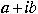. Such interpretation of the moment is broader than the classical one.

Configuration vectors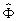and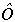,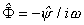and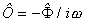(2.14)

correspond to the harmonic displacement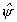.

2.3. A potentially-kinetic speed of harmonic displacement

The time derivative of the binary displacement defines the contradictory potentially-kinetic velocity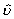of displacement: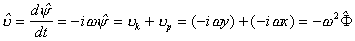. (2.15)

The first component of the velocity that characterizes motion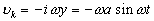(2.16)

will be called kinetic velocity and the second component that describes rest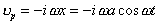(2.17)

will be called potential velocity.

The kinetic velocity defines the intensity of motion and is an ordinary velocity of displacement of an object in space. The larger the kinetic velocity, the higher the degree of non-occurrence of the object at the point.

The potential velocity defines the intensity of rest and the larger the velocity, the higher the degree of occurrence of the object at the point. The potential velocity is a new concept and we will gradually fill it with concrete contents.

The field of potential-kinetic velocitydescribes the first-level field of rest-motion, the field of displacement.

The specific displacement defines the specific velocity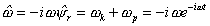. (2.18)

2.4. Components of the field of potentially-kinetic acceleration

The field of potential-kinetic acceleration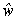describes the second-level field of rest-motion, the velocityfield: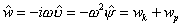. (2.19)

The first component of the acceleration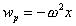(2.20)

will be named potential acceleration and the second component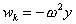(2.21)

kinetic acceleration.

The derivative of specific velocity defines the specific acceleration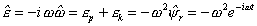, (2.22)

where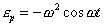(2.22a)

is the potential component of the specific acceleration and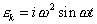(2.22b)

is the kinetic component of it.

2.5. Multilevel nature of the rest-motion field

In a general case, it is reasonable to operate with the derivatives of any orders which describe the fields of motion-rest states, expressed by the potential-kinetic lower-order derivatives.

The field of state defined by a state vector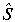together with derivative fields is a multilevel field of motion-rest.

Each from the levels is an individual field of motion-rest. The number of levels is usually infinite, in this sense a rest-motion field is irrational. If we restrict ourselves by a finite number of levels, we should speak about a rational field of rest-motion. In a general case, the field of motion-rest has contradictory rational-irrational nature.

In relation to the overlying field, any arbitrary-level parameter of rest-motion is passive, reactive in the sense that it is a definite measure-oppositus of the state of this level. At the same time, it is active in relation to the underlying field because it describes motion-rest exchange on this level.

2.6. The rest-motion field of kinetic-potential momentum

To the state vector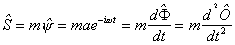(2.23)

corresponds to the specific state vector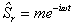. (2.23a)

As a scalar, the specific state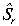will be called the potential-kinetic mass m: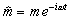. (2.24)

The first derivative of state S defines the field of motion-rest of the kinetic-potential momentum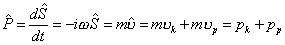(2.25)

or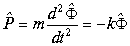, (2.26)

where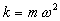is rigidity of the state.

The first component of the momentum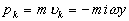(2.27)

is a kinetic momentum; the second component of the momentum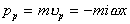(2.28)

is a potential momentum.

The derivative of the specific stateor potential-kinetic mass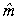defines the specific momentum or kinetic-potential charge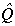: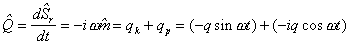, (2.29)

where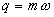is the modulus of the kinematic charge;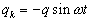is a kinetic charge;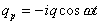is a potential charge.

The moment of momentum is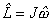, (2.30)

where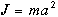is a moment of inertia of harmonic oscillation

2.7. Potential-kinetic kinema

The field of momentum has its field of motion-rest, which is determined by the second derivative of state or the first derivative of momentum. The vector of this field will be called a kinema: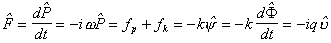. (2.31)

The first component of the kinema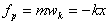(2.32)

is a potential kinema: the second component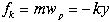(2.33)

is a kinetic kinema. The specific kinema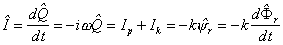(2.34)

will be named potential-kinetic or kinematic current.

The potential-kinetic kinema, as a field of state, describes the state by itself of a material point on this level and it is inactive. It cannot be identified with the force in Newton's mechanics. On the other hand, it is active, relative to the field of momentum and in this sense the potential-kinetic kinema describes the rest-motion exchange of the field of momentum.

The potential kinema, as a measure of exchange, expresses, to some extent, a human subjective sensation of exchange, which can be defined as sensation of "elasticity" of rest. The kinetic kinema provides a sensation of "elasticity" of motion i.e. the larger motion speed the harder change a motion state.

We will define the moment of kinema by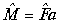.(2.35)

The moment of momentum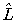and the moment of kinema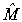are related by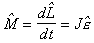. (2.36)

similar to the corresponding relation in the theory of rotational motion.

2.8. Description of a field of variation of kinema by mobilite

The time derivative of kinema defines a potential-kinetic field of change of the field of kinema. This derivative will be named a mobilite (from the Latin, mobilita=mobility):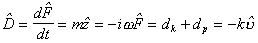, (2.37)

where z is the acceleration velocity. The first component of the mobilite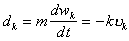(2.38)

is a kinetic mobilite and the second component of the mobilite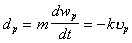(2.39)

is a potential mobilite.

On the one hand, the potential-kinetic mobilite describes the field of motion of the kinema and is a characteristic of this exchange. On the other hand, it expresses the state of a material point, which is proportional to the field of velocity while harmonic oscillation.

Theoretical Dialectical Journal: Physics-Mathematics-Logic-Philosophy, N.2, site http://www.tedial.narod.ru/